# From FFT preview

Select Channel

Set Sample rate eg. 50kHzSet FFT options to:

• DC Cutoff 10Hz
• Line resolution 8192 lines (df=3.05 Hz should be lower than 10Hz !)
• Amplitude display RMS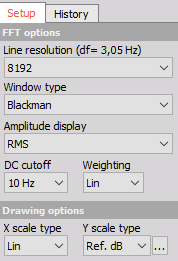Set Drawing options to:

• Y scale type Ref. dB
• dB scaling reference point = Measurement range / sqrt(2), eg 35.5Vrms for 50V MR.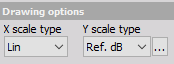Scale X axis from 0Hz to Sample rate/2, eg. 0 .. 25000 Hz for SR = 50000 HzOn FFT preview window select RMS in top right corner.

RMS values and (zoomed) should be the same!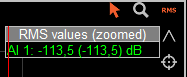# From Math

Select Basic stat. and select Output channels:

• RMS
• AverageSetup formula for AC RMS:

• sqrt(sqr('AI 1/RMS')-sqr('AI 1/AVE'))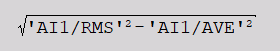Setup formula for Noise floor:

• 20*log10('AC RMS'/('MR'/sqrt(2)))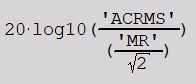Result of the formula and FFT preview should be identical:When using FFT approach be careful when setting Scale on X (frequency) axis, depending on this (zoomed) value will change.

Careful with DC cutoff and Line resolution:

• df=3.05Hz should be lower than DC cutoff freq 10Hz.• component near DC will influence the Noise floor result.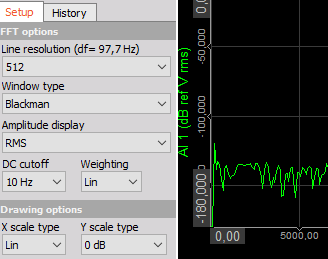Y scale type: dB scaling reference point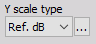• 50 V equals 3dB
• 35.3 V equals 0dB

Both of the above will give identical results.

Using Y scale type: 0dB will add additional -3dB to the result.

• eg -113dB for Ref. dB type (and MR/sqrt(2))
• eg. -116dB for 0dB type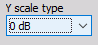Correct this result with math forumula:

• 20*log10('AC RMS'/('MR'/sqrt(2)))-3Instead of formula for AC RMS calculation one can use HP filtering in order to eliminate DC component and use math to calculate RMS value which is by design now AC RMS, then calculate Noise in dB as before.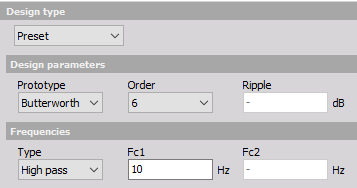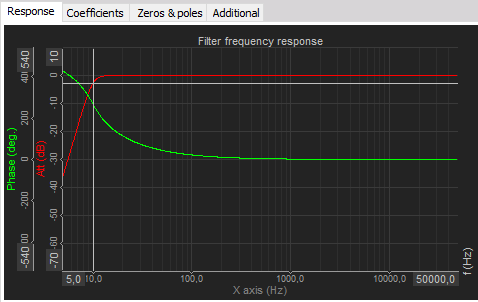Jernej Kovačič, 11.6.2020